# WBJEE 2018 Physics Paper with Solutions

WBJEE 2018 Paper with Solutions Physics is available here. Revise them and gain confidence, which in turn reduces the fear of facing the examination. It will help the students to attempt maximum questions in the stipulated time by increasing their speed and accuracy. Candidates can download the previous years’ question papers of WBJEE to figure out the alternative and easy method to solve a particular problem. Practising the previous year papers is the best tool for the preparation of this competitive exam WBJEE, in terms of the syllabus and distribution of marks across different topics. Solving these papers with solutions will boost the candidates’ confidence and scores in the WBJEE examination.

### WBJEE 2018 - Physics

Category - I (Q.1 to Q.30)

Carry 1 mark each and only one option is correct. In case of incorrect answer or any combination of more than one answer, ¼ mark will be deducted.

Question 1: The velocity (v) of a particle (under a force F) depends on its distance (x) from the origin (with x > 0) v ∝ (1 / √x). Find how the magnitude of the force (F) on the particle depends on x.

1. a. F ∝ 1 / x3/2
2. b. F ∝ 1 / x
3. c. F ∝ 1 / x2
4. d. F ∝ x

Solution:

Given that, v ∝ (1 / √x)

On differentiating with respect to time ‘t’.

$$a = \frac{dv}{dt} = \frac{dv}{dt} \times \frac{dx}{dx} = v \times \frac{dv}{dx} = v \times \frac{d}{dx}(\frac{k}{\sqrt{x}})$$

$$a=\frac{-k^2}{2\sqrt{x}(x)^{3/2}} =\frac{-k^2}{2x^2}$$

F ∝ 1 / x2

Question 2: The ratio of accelerations due to gravity g1: g2 on the surfaces of two planets is 5:2 and the ratio of their respective average densities 𝞀1:𝞀2 is 2:1. What is the ratio of respective escape velocities v1: v2 from the surface of the plants?

1. a. 5 : 2
2. b. √5 : √2
3. c. 5 : 2 √2
4. d. 25 : 4

Solution:

Given that, g1 / g2 = 5 / 2

The ratio of their respective average densities 𝞀1 / 𝞀2 = 2 / 1

Now, we have to calculate the ratio of respective escape velocities v1: v2 from the surface of planets.

We know that

-Gmm / R + (1 / 2)mv2 = 0

v = √(2GM/R) = √[(2GM / R2) * R] = √2GR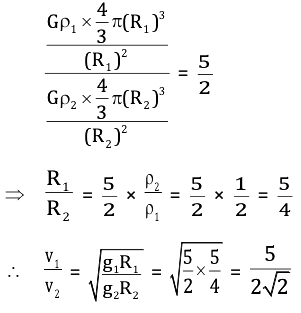Question 3: A spherical liquid drop is placed on a horizontal plane. A small disturbance causes the volume of the drop to oscillate. The time period of oscillation (T) of the liquid drop depends on the radius (r) of the drop, density (𝞀) and surface tension (s) of the liquid. Which among the following will be a possible expression for T (where k is a dimensionless constant)?

1. a. k √𝞀r / s
2. b. k √𝞀2r / s
3. c. k √𝞀r3 / s
4. d. √𝞀r3 / s2

Solution:

Now, according to the question, we can write the equation.

T = Krx𝞀ysz

T → T, r → L, 𝞀 → ML–3, s → MT–2

S = F / l = ma / l = m / s2

T1 = KLx (ML–3)y (MT–2)z

T1 = KLx-3y M(y+z)T(-2z)

–2z = 1

z = – 1 / 2 , y + z = 0, y = –z = + 1 / 2

x – 3y = 0

x = 3y = 3 / 2

T = K63/2𝞀1/2s = K √(𝞀r3/s)

Question 4: The stress along the length of a rod (with rectangular cross-section) is 1% of Young's modulus of its material. What is the approximate percentage of change of its volume? (Poisson's ratio of the material of the rod is 0.3)

1. a. 3%
2. b. 1%
3. c. 0.7%
4. d. 0.4%

Solution:

We know that, v = xyz

(dv / v) = (dx / x) + (dy / y) + (dz / z)

(dl / l) - μ (dl / l) - μ (dl / l) = (1 - 2μ) (dl / l)

Now, we know that

Stress = y × strain

0.01y = y * (dl / l)

(dl / l) = 0.01

So, (dv / v) = 0.01 * (1 - 0.6) = 0.01 × 0.4

Percentage volume change is = 0.4%

Question 5: What will be the approximate terminal velocity of a raindrop of diameter 1.8 × 10–3 m, when the density of rainwater ≈ 103 kgm–3 and the coefficient of the viscosity of air ≈ 1.8 × 10–5 Nsm–2? (Neglect buoyancy of air).

1. a. 48 ms–1
2. b. 98 ms–1
3. c. 392 ms–1
4. d. 980 ms–1

Solution:

We know that,

6𝛑𝛈rv = (4 / 3) 𝛑r3𝞀g

v = 2 / [9𝛈r2𝞀g] = [2 * 0.9 * 0.9 * 10-6 * 103 * 9.8] / [9 * 1.8 * 10-5]

v = 9.8 × 10–6 × 107 = 98 m/sec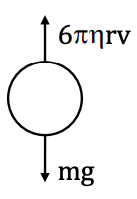Question 6: The water equivalent of a calorimeter is 10 g and it contains 50 g of water at 15°C. Some amount of ice, initially at –10°C is dropped in it and half of the ice melts till equilibrium is reached. What was the initial amount of ice that was dropped (when specific heat of ice = 0.5 cal gm–1°C–1, specific heat of water = 1.0 cal gm–1°C–1 and latent heat of melting of ice = 80 cal gm–1)?

1. a. 10 g
2. b. 18 g
3. c. 20 g
4. d. 30 g

Solution: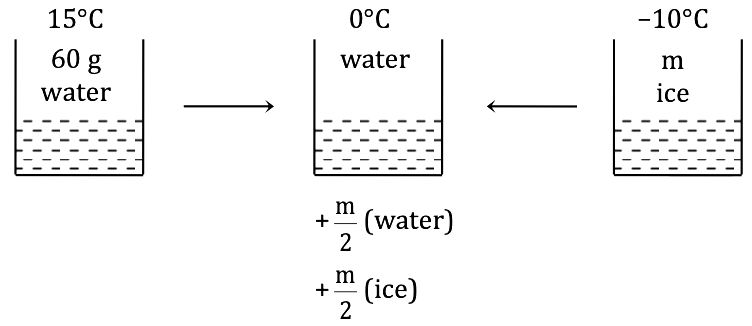m × 1 / 2 × 10 + [m / 2] × 80 = 60 × 1 × 15

m = [60 * 15] / 45 = 20 gm

Question 7: One mole of a mono-atomic ideal gas undergoes a quasi-static process, which is depicted by a straight line joining points (V0, T0) and (2V0, 3T0) in a V-T diagram. What is the value of the heat capacity of the gas at the point (V0, T0)?

1. a. R
2. b. (3 / 2) R
3. c. 2R
4. d. 0

Solution:

According to the question, we can draw its (V–T) graph.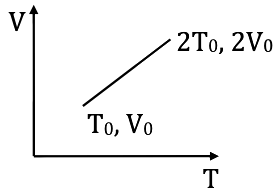Now, we can write

Pdv + nCVdT = ncdT

C = CV + (p / n) (dv / dT)

At, V0, T0

dv / dT = V0 / 2T0, P0V0 = nRT0

C = (3 / 2)R + [(RT0 + 0) / V0] * (V0 / 2T0) = (3 / 2)R + (R / 2) = 2R

Question 8: For an ideal gas with initial pressure and volume Pi and Vi, respectively, a reversible isothermal expansion happens, when its volume becomes V0. Then it is compressed to its original volume Vi by a reversible adiabatic process. If the final pressure is Pf then which of the following statements is true?

1. a. Pf = Pi
2. b. Pf > Pi
3. c. Pf < Pi
4. d. Pf / V0 = Pi / Vi

Solution:

Now, according to the question, we can draw (P-V) graph.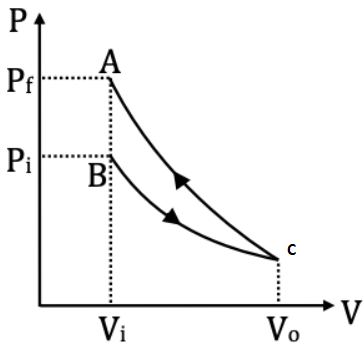There is a reversible adiabatic process in the curve AC and isothermal process in the curve BC. Now, from the graph, we can say that pf > pi.

Question 9: A point charge – q is carried from a point A to another point B on the axis of a charged ring of radius ‘r’ carrying a charge +q. If the point A is at a distance (4 / 3) r from the centre of the ring and the point B is (3 / 4) r from the centre but on the opposite side, what is the network that needs to be done for this?

1. a. (-7 / 5) (q2 / 4𝛑𝝐0r)
2. b. (-1 / 5) (q2 / 4𝛑𝝐0r)
3. c. (7 / 5) (q2 / 4𝛑𝝐0r)
4. d. (1 / 5) (q2 / 4𝛑𝝐0r)

Solution:

We know that,

W = UB = UA

W = -Kqq / √[r2 + (9 / 16) r2] + Kq2 / [r2 + (16 / 9) r2]

W = (-4 / 5) (Kq2 / r) + (3 / 5) (Kq2 / r) = -Kq2 / 5r

W = (-1 / 5) (q2 / 4𝛑𝝐0r)

Question 10: Consider a region in free space bounded by the surfaces of an imaginary cube having sides of length ‘a’ as shown in the diagram. A charge +Q is placed at the centre ‘O’ of the cube. P is such a point outside the cube that the line OP perpendicularly intersects the surface ABCD at R and also OR = RP = a / 2. A charge +Q is placed at point P also. What is the total electric flux through the five faces of the cube other than ABCD?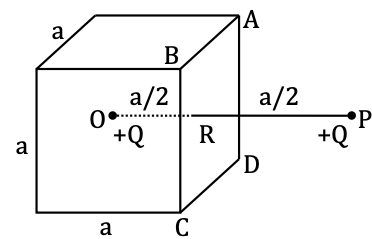1. a. Q / 𝝐0
2. b. 5Q / 6𝝐0
3. c. 10Q / 6𝝐0
4. d. Zero

Solution:

Flux due to charge at 0

Now, for five faces of cube other than ABCD,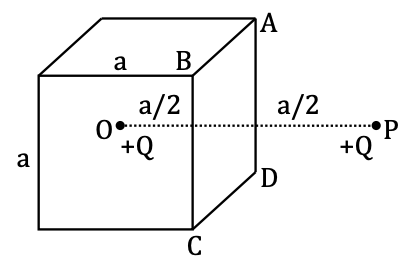ɸ1 = 5 × (Q/(6𝝐0))

Flux due to charge at P, through face ABCD will be equal to through all other five faces.

ɸ2 = (Q/(6𝝐0))

ɸnet = ɸ1 + ɸ2 = Q/(𝝐0)

Question 11: Four equal charges of value +Q are placed at any four vertices of a regular hexagon of side ‘a’. By choosing the vertices, what can be the maximum possible magnitude of the electric field at the centre of the hexagon?

1. a. Q / [4𝛑𝝐0a2]
2. b. √2 / [4𝛑𝝐0a2]
3. c. (√3Q) / [4𝛑𝝐0a2]
4. d. 2Q / [4𝛑𝝐0a2]

Solution:

Now, according to the questions, we will draw a regular hexagon of side ‘a’. According to the question, on distributing four charges at any four vertices of a regular hexagon of side ‘a’.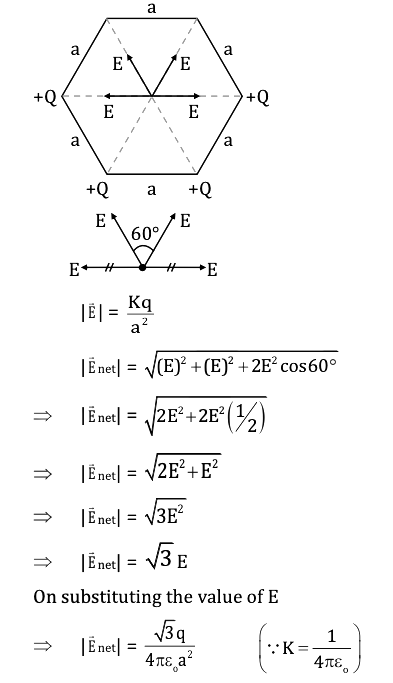Question 12: A proton of mass 'm' moving with a speed v (<< c, the velocity of light in vacuum) completes a circular orbit in time 'T' in a uniform magnetic field. If the speed of the proton is increased to 2 v, what will be the time needed to complete the circular orbit?

1. a. √2 T
2. b. T
3. c. T / √2
4. d. T / 2

Solution:

Since the time period (T) of a photon will be given by

T = 2πm / qB

We can see that the time period is independent of the velocity/speed.

So, we can say that there will be no change in the time period of the photon.

Question 13: A uniform current is flowing along the length of an infinite, straight, thin, hollow cylinder of radius 'R'. The magnetic field 'B' produced at a perpendicular distance 'd' from the axis of the cylinder is plotted in a graph. Which of the following figures looks like the plot?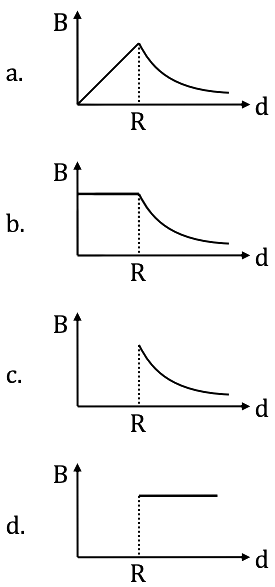Solution: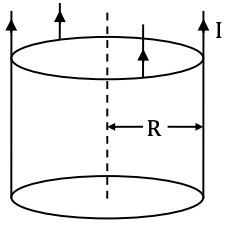From the above figure, we can see that

For d < R,

B × 2πd = µoIin = 0

B = 0

For d > R,

B × 2πd = µoI

B = µ0I / 2πd

Question 14: A circular loop of radius 'r' of conducting wire connected with a voltage source of zero internal resistance produces a magnetic field 'B' at its centre. If instead, a circular loop of radius '2r' made of the same material, having the same cross-section is connected to the same voltage source, what will be the magnetic field at its centre?

1. a. B / 2
2. b. B / 4
3. c. 2B
4. d. B

Solution:

When we will double its radius, the resistance in the circuit will also be doubled. When resistance in the circuit will double, then current in the circuit will be decreased by halved.

B = (µoI / 2r)

B’ = (µoI’ / 2r’) [∵ I’ = I / 2, r’ = 2r]

B’ = (µoI / 8r)

B’ = B / 4

Question 15: An alternating current is flowing through a series LCR circuit. It is found that the current reaches a value of 1 mA at both 200 Hz and 800 Hz frequency. What is the Resonance frequency of the circuit?

1. a. 600 Hz
2. b. 300 Hz
3. c. 500 Hz
4. d. 400 Hz

Solution:

Since according to the questions, we can write.

ωL = 1 / ωC [∵ ω = 2𝛑f and f = 1 / [2𝛑 √LC]]

ω2 = 1 / LC

Squaring both sides, we get

ω = √(1 / LC)

XL and XC will get interchanged.

200L = [1 / 800] C

√(1 / LC) = √(200 * 800) = 400 Hz

Question 16: An electric bulb, a capacitor, battery and a switch are all in series in a circuit. How does the intensity of light vary when the switch is turned on?

1. a. Continues to increase gradually.
3. c. Sharply rises initially and then gradually decreases.

Solution:

Initially, there will be no voltage drop across the capacitor so the intensity of bulb will rise sharply and gradually voltage drop across the capacitor will increase as a result voltage drop across bulb decreases so the intensity of bulb will decrease.

Question 17: Four resistors, 100 Ω, 200 Ω, 300 Ω, and 400 Ω, are connected to form four sides of a square. The resistors can be connected in any order. What is the maximum possible equivalent resistance across the diagonal of the square?

1. a. 210 Ω
2. b. 240 Ω
3. c. 300 Ω
4. d. 250 Ω

Solution:

According to the question, the circuit diagram will be given by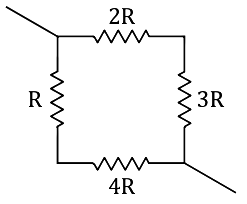Now, we can write the maximum possible equivalent resistance across the diagonal of the square.

Reff = [5R × 5R] / [5R + 5R]

= 25R2 / 10R

= 25R / 10

= [25 * 100] / 10

= 250 Ω

Question 18: What will be current through the 210 Ω resistor in the given circuit a long time after the switch 'K' is made on?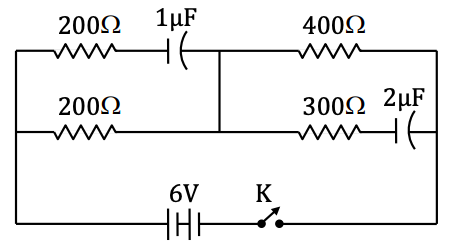1. a. Zero
2. b. 100 mA
3. c. 10 mA
4. d. 1 mA

Solution:

After a long time, when the switch is on, then it will become steady-state and in steady-state, the capacitor will behave as infinite resistance. The steady current will be given by

Isteady = V / R = 6 / 600 = 10mA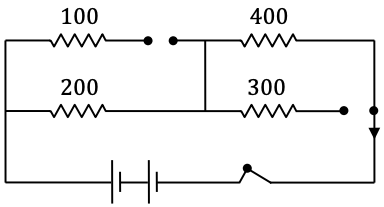Question 19: A point source is placed at coordinates (0, 1) in the X-Y plane. A ray of light from the source is reflected on a plane along the X-axis and perpendicular to the X-Y plane. The reflected ray passes through the point (3, 3). What is the path length of the ray from (0, 1) to (3, 3)?

1. a. 5
2. b. √13
3. c. 2 √13
4. d. 1+ 2 √13

Solution: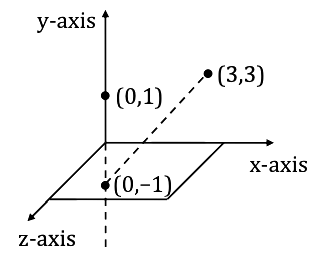Magnitude of ‘a’ will be given by

|a| = √(32 + 42) = 5

|a| = 5

Question 20: Two identical equi-convex lenses, each of focal length 'f' are placed side by side in contact with each other with a layer of water in between them as shown in the figure. If the refractive index of the material of the lenses is greater than that of water, how the combined focal length 'F' is related to 'f'?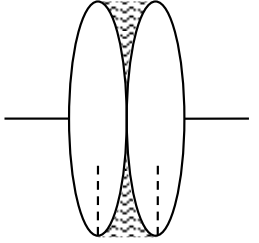1. a. F > f
2. b. [f / 2] < F < f
3. c. F < [f / 2]
4. d. F = f

Solution:

To combine the equi-convex lenses, such that (F) is related to (f)-

Now, according to formula,

(1 / fw) = (μw - 1) (-2 / R) = {[μw - 1] / [μl - 1]} [1 / fl]

Now, [1 / fl] = (μl - 1) (2 / R)

μl > μw

Now, [1 / feq] = [2 / fl] - [1 / fl] [(μw - 1) / (μl - 1)]

l - 1) > (μw - 1)

[(μw - 1) / (μl - 1)] < 1

(1 / f) < (1 / feq) < (2 / f)

(2 / f) < feq < f

Question 21: There is a small air bubble at the centre of a solid glass sphere of radius 'r' and refractive index 'µ'. What will be the apparent distance of the bubble from the centre of the sphere, when viewed from outside?

1. a. r
2. b. r / µ
3. c. r [1 - (1 / µ)]
4. d. Zero

Solution: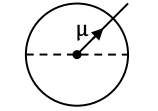As the object is at the centre, all rays will fall normally on the surface, hence they will not deviate.

Apparent depth = Real depth

Question 22: If Young's double-slit experiment is done with white light, which of the following statements will be true?

1. a. All the bright fringes will be coloured.
2. b. All the bright fringes will be white.
3. c. The central fringe will be white.
4. d. No stable interference pattern will be white.

Solution:

If Young’s double-slit experiment is done with white light, then the centre fringe will be white because Δx = 0 at the centre for all the wavelengths.

Question 23: How the linear velocity 'v' of an electron in the Bohr orbit is related to its quantum number 'n'?

1. a. v ∝ 1 / n
2. b. v ∝ 1 / n2
3. c. v ∝ 1 / √n
4. d. v ∝ n

Solution:

The linear velocity ‘v’ of on electron in the Bohr orbit is related to its quantum number (n) will be given by

v = ze2 / 2𝝐0nh

Question 24: If the half-life of a radioactive nucleus is 3 days, nearly what fraction of the initial number of nuclei will decay on the 3rd day? (Given that ∛0.25 ≈ 0.63)

1. a. 0.63
2. b. 0.5
3. c. 0.37
4. d. 0.13

Solution:

Fractional decay on the third day will be given by-

= [N0e-2/τ - N0e-3/τ] / N0

τ = t1/2 / ln 2 = 3 / ln 2

= e-2ln2/3 - e-ln2

= 2-⅔ - 2-1

= 0.63 – 0.5

= 0.13

Question 25: An electron accelerated through a potential of 10,000 V from rest has a de-Broglie wavelength “λ''. What should be the accelerating potential so that the wavelength is doubled?

1. a. 20,000 V
2. b. 40,000 V
3. c. 5,000 V
4. d. 2,500 V

Solution:

Since we know that

Ve = hc / λ

(10000)e = hc / λ

hc / 2λ = (5000) e

Question 26: In the circuit shown, inputs A and B are in states ‘1’ and ‘0’ respectively. What is the only possible stable state of the outputs ‘X’ and ‘Y’?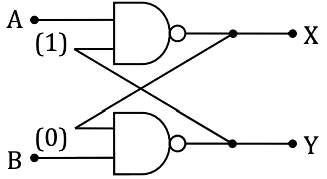1. a. X = ‘1’, ‘Y’ = ‘1’
2. b. X = ‘1’, ‘Y’ = ‘0’
3. c. X = ‘0’, ‘Y’ = ‘1’
4. d. X = ‘0’, ‘Y’ = ‘0’

Solution: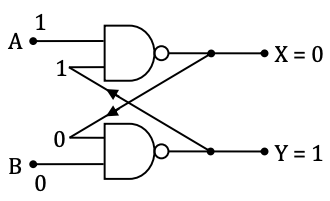Firstly checking this from output side

When X = 0 and Y = 1

And A = 1 and B = 0 is given so

By A.B = AND GATE

0.0 = 0

1.1 = 1

Question 27: What will be the current flowing through the 6KΩ resistor in the circuit shown, where the breakdown voltage of the Zener is 6 V?1. a. (2 / 3) mA
2. b. 1 mA
3. c. 10 mA
4. d. (3 / 2) mA

Solution: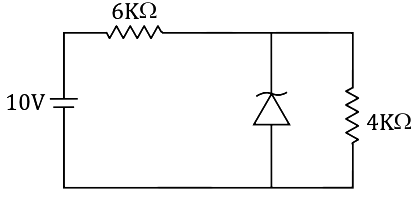Zener break done = 6V

So potential across 4KΩ = 6V

And potential across 6KΩ = (10 – 6) = 4V

Current through the 6KΩ = [4 / 6000] A

[2 / 3000] A = [2 / 3] mA

Question 28: In case of a simple harmonic motion, if the velocity is plotted along the X-axis and the displacement (from the equilibrium position) (is plotted along the Y-axis, the resultant curve happens to be an ellipse with the ratio:

major axis (along X) / minor axis (along Y) = 20𝛑

What is the frequency of the simple harmonic motion?

1. a. 100 Hz
2. b. 20 Hz
3. c. 10 Hz
4. d. (1 / 10) Hz

Solution:

The relation between velocity (v) and displacement (x) in SHM is H.

[v2 / w2A2] - [x2 / A2] = 1 [It is equation of Ellipse]

Elliptical path having radius equal to (a)

Major axis will be = 2wA

And minor axis will be = 2a

Given: 2ωa / 2a

ω = 20𝛑

2𝛑f = 20𝛑

f = 10 Hz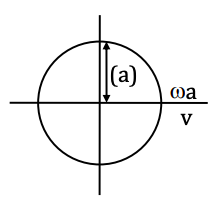Question 29: A block of mass m2 is placed on a horizontal table and another block of mass m1 is placed on top of it. An increasing horizontal force F = αt is exerted on the upper block but the lower block never moves as a result. If the coefficient of friction between the blocks is µ1 and that between the lower block and the table is µ2, then what is the maximum possible value of µ1 / µ2?

1. a. m2 / m1
2. b. 1 + [m2 / m1]
3. c. m1 / m2
4. d. 1 + [m1 / m2]

Solution:

Now, according to the question, we can draw the figure.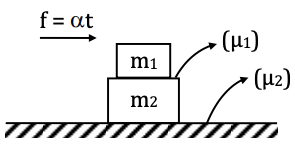1) is the coefficient of friction between the block (m1) and (m2). And (µ2) is the coefficient of friction between the block (m2) and the surface.

Now, F.B.D. of the lower block (m2)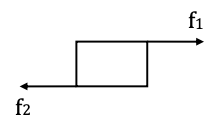(f2) is the friction between the lower block and the table and (f1) is the friction between the lower block and upper block.

m2 is not moving.

So, f1 ≤ f2

So, friction acting between the block is always less than or equal to friction acting between lower block and table.

So, µ1m1g ≤ µ2 (m1 + m2) g

µ1 / µ2 = [m1 + m2] / m1

µ1 / µ2 ≤ 1 + (m2 / m1)

So, maximum value of µ1 / µ2 = 1 + (m2 / m1)

Question 30: In a triangle ABC, the sides AB and AC are represented by the vectors

$$3\hat{i} + \hat{j} + \hat{k}$$
and
$$\hat{i} + 2\hat{j} + \hat{k}$$
respectively. Calculate the angle ABC.

1. a. cos–1 √(5/11)
2. b. cos–1 √(6/11)
3. c. 90o – cos-1 √(5/11)
4. d. 180o – cos-1 √(5/11)

Solution:

Vectors, AB and AC are given

According to the question

Vector CB will be given by

$$\vec{CB} = \vec{AB} – \vec{AC}$$

$$\vec{CB} = 2\hat{i} – \hat{j}$$

Now, B is between AB and CB.

So, it will be given by the dot product of two vectors.

$$\vec{AB} . \vec{CB} = | \vec{AB} | | \vec{CB} | cos \theta$$

| AB | = √32 + 12 +12 = 11

And | CB | = √22 + (-1)2 = 5

$$\vec{AB} . \vec{CB}$$
= | √11 | | √5 | cos θ

cos θ = √5 / √11

θ = cos–1 √(5/11)

Category - II (Q.31 to Q.35)

Carry 2 marks each and only one option is correct. In case of incorrect answer or any combination of more than one answer, ½ mark will be deducted.

Question 31: The insulated plates of a charged parallel plate capacitor (with a small separation between the plates) are approaching each other due to electrostatic attraction. Assuming no other force to be operative and no radiation taking place, which of the following graphs approximately shows the variation with time (t) of the potential difference (V) between the plates?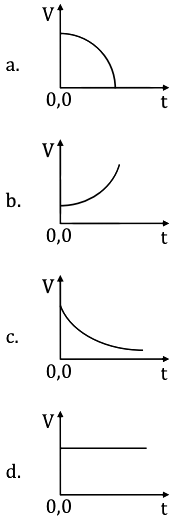Solution: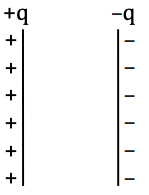As the separation between the plates is decreasing as they are approaching each other.

Also, V = E.d

Now, the electric field will remain constant between the plates.

So, V ∝ d

Now, the force on each plate will be = q2 / 2A𝝐0

And acceleration = q2 / 2A𝝐0m

∵ a = constant so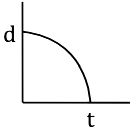So, (v-t) curve will be given by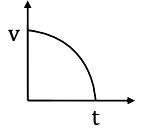Question 32: The bob of a pendulum of mass 'm', suspended by an inextensible string of length 'L' as shown in the figure carries a small charge 'a'. An infinite horizontal plane conductor with uniform surface charge density σ is placed below it. What will be the time period of the pendulum for small amplitude oscillations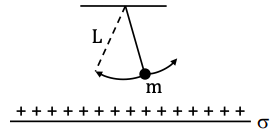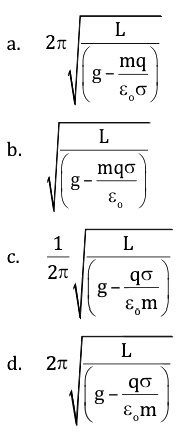Solution:

Force on the bob of the pendulum of mass (m) will be given by

F = qE

F = ma

ma = qE

a = qE / m

Now, time period (T) of a simple pendulum will be given by

T = 2𝛑 √ L / g'

g’ = g – a

T = 2𝛑 √L / (g - [qE / m])

T = 2𝛑 √L / {g - [qσ / 𝝐0m]}

Question 33: A light charged particle is revolving in a circle of radius 'r' in the electrostatic attraction of a static heavy particle with opposite charge. How does the magnetic field 'B' at the centre of the circle due to the moving charge depends on 'r'?

1. a. B ∝ 1 / r
2. b. B ∝ 1 / r2
3. c. B ∝ 1 / r3/2
4. d. B ∝ 1 / r5/2

Solution: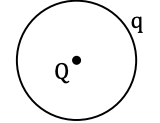The electrostatic force of attraction will be given by

F = KqQ / r2

Since the charge is in a circular motion, it will experience centripetal force

F = mv2/ r

[mv2/ r] = [KqQ / r2]

mv2 = KQq / r

v = √(KqQ/rm)

v ∝ 1 / √r

Time period = 2𝛑r / v

Time period ∝ [r / v]

Time period ∝ r3/2

I ∝ Q / time period [∴ I ∝ r-3/2]

B = μ0I / 2r

B ∝ I or B ∝ I / r

B ∝ I / r or B ∝ r-3/2 / r

B ∝ r-5/2

Question 34: As shown in the figure, a rectangular loop of a conducting wire is moving away with a constant velocity 'v' in a perpendicular direction from a very long straight conductor carrying a steady current 'I'. When the breadth of the rectangular loop is very small compared to its distance from the straight conductor, how does the e.m.f. 'E' induced in the loop varies with time 't'?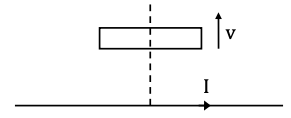1. a. E ∝ (1 / t2)
2. b. E ∝ (1 / t)
3. c. E ∝ ln(t)
4. d. E ∝ 1 / t3

Solution:

Since we know that

𝝐 = -dɸ / dt

= - d [B . A] / dt

= -A [dB / dt]

= -A (d / dt) (μ0I / 2𝛑 (vt)) [∵ B = (μ0I / 2𝛑 (vt))]

= -A (μ0I / 2𝛑v) (d / dt) (t-1)

After differentiating,

= A (μ0I / 2𝛑v) t-2

E ∝ (1 / t2)

Question 35: A solid spherical ball and a hollow spherical ball of two different materials of densities 𝞀1 and 𝞀2 respectively have the same outer radii and the same mass. What will be the ratio of the moment of inertia (about an axis passing through the centre) of the hollow sphere to that of the solid sphere?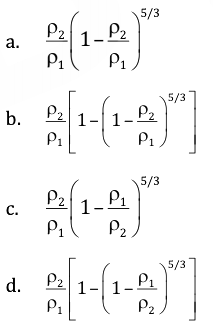Solution:

According to question

𝞀1 → Solid spherical ball

𝞀2 → Hollow spherical ball

Now,

μ = (4 / 3) 𝛑𝞀1R3 = (4 / 3) 𝛑𝞀2 [R3 - R3inner]

[R3 - R3inner] = 𝞀1R3/𝞀2

R3inner = R3 [1 - (𝞀1 / 𝞀2)]

Rinner = R (1 - (𝞀1 / 𝞀2))

The ratio of the moment of inertia will be: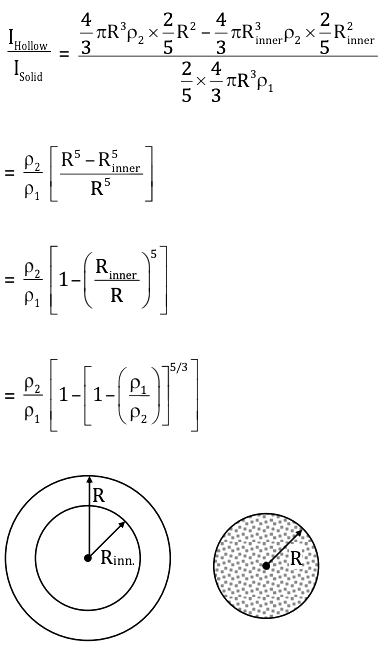Category - III (Q.36 to Q.40)

Carry 2 marks each and one or more option(s) is/are correct. If all correct answers are not marked and also no incorrect answer is marked then score = 2 × number of correct answers marked the actual number of correct answers. If any wrong option is marked or if any combination including a wrong option is marked, the answer will be considered wrong but there is no negative marking for the same and zero marks will be awarded.

Question 36: Which of the following statement(s) is/are true? "Internal energy of an ideal gas ………………"

1. a. Decreases in an isobaric process.
2. b. Remains constant in an isothermal process.
3. c. Increases in an isobaric process.
4. d. Decreases in an isobaric expansion.

Solution:

As we know, that in the isothermal process the change in internal energy is always zero and in isobaric expansion, the volume is directly proportional to temperature. So, the change in internal energy will increase.

Question 37: Two positive charges Q and 4Q are placed at points A and B respectively, where B is at a distance 'd' units to the right of A. The total electric potential due to these charges is minimum at P on the line through A and B. What is (are) the distance(s) of P from A?

1. a. (d / 3) units to the right of A
2. b. (d / 3) units to the left of A
3. c. (d / 3) units to the right of A
4. d. d units to the left of A

Solution:

As we know, the electric potential will be minimum, where the electric field will be zero. Now, let us assume that the charge Q is at (x) distance from point P as well as charge 4Q is at (d–x) distance from point P.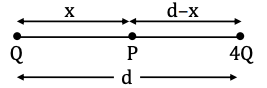The electric potential due to charge (Q) at point P will be

EP = KQ / x2

Similarly, the electric potential due to charge (4Q) at point P will be:

EP = K4Q / (d - x)2

On equating,

KQ / x2 = K4Q / (d - x)2

1 / x2 = 4 / (d - x)2

(1 / x)2 = (2 / [d - x])2

1 / x = 2 / (d - x)

x = d / 3

Electric potential will be minimum at (d / 3) units, at right of point A.

Question 38: A non–zero current passes through the galvanometer G shown in the circuit when the key 'K' is closed and its value does not change when the key is opened. Then which of the following statement(s) is/are true?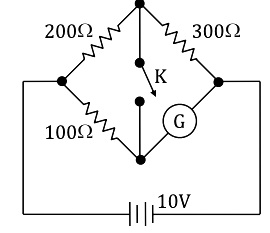1. a. The galvanometer resistance is infinite.
2. b. The current through the galvanometer is 40 mA.
3. c. After the key is closed, the current through the 200 Ω resistor is the same as the current through the 300 Ω resistor.
4. d. The galvanometer resistance is 150.

Solution:

When switch will be opened then it will be a case of balanced wheat-stone bridge 200 / 300 = 100 / a

G = 150 Ω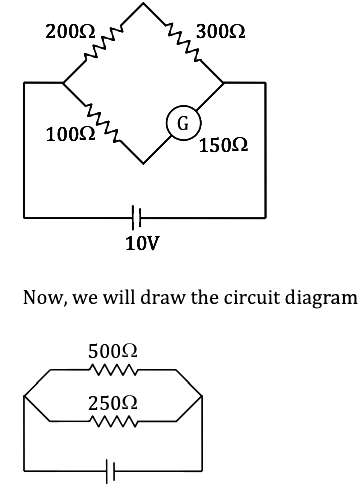Req. = [500 * 250] / 750 = (500 / 3) Ω

Now, current through the galvanometer

10 / 250 = 1 / 25 = 0.04 A

200 Ω and 300 Ω are in series.

100 Ω, 150 Ω and (G) are in series.

Question 39: A ray of light is incident on a right-angled isosceles prism parallel to its base as shown in the figure. Refractive index of the material of the prism is √2. Then which of the following statement(s) is/are true?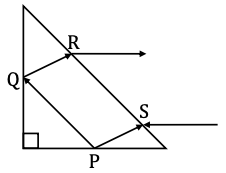1. a. The reflection at P is totally internal.
2. b. The reflection at Q is totally internal.
3. c. The ray emerging at R is parallel to the ray incident at S.
4. d. Total deviation of the ray is 150°.

Solution:

Applying geometry to the given figure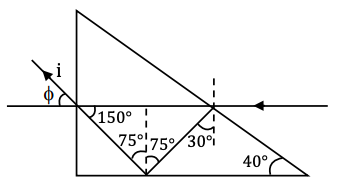On applying Snell’s law in the given figure

1 sin(i) = √2 sin 150

1 sin(i) = √2 {[√3 - 1] / [2√2]}

sin(i) = {[√3 - 1] / }

This ray will go under ‘total internal reflection’ at point P only. So, option (a) is correct.

If the ray emerges from R (partially) it is possible, then it will become parallel to the incident ray. So, option (c) is correct.

Question 40: The intensity of a sound appears to an observer to be periodic. Which of the following can be the cause of it?

1. a. The intensity of the source is periodic.
2. b. The source is moving towards the observer.
3. c. The observer is moving away from the source.
4. d. The source is producing a sound composed of two nearby frequencies.

Solution:

Due to the difference in frequencies, the phenomenon of beat occurs.

### WBJEE 2018 Physics Question Paper with Solutions